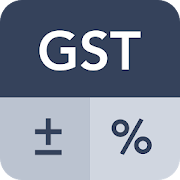# GST Calculator - ToolEveryone
4,592
Calcuate GST Tax on the go along with other mathematical calculations. GST Calculator Tool is simple, easy to use and the quickest way to calculate Goods and Service Tax (GST) on sales or purchase of goods or services.

GST Calculator Tool is combination of two calculators:

## Simple GST Calculator

Useful to calculate GST quickly with simple interface optimised for GST calculations.

Simply enter the Initial Amount of transaction and the GST Rate and get the results calculated as you type in. You can see the amount inclusive or exclusive of tax amount. Simply use "Add GST" to get the total amount of goods or services including GST or "Remove GST" to get the net amount of goods or services exclusive of GST.

Generally kept GST calculations as a center point, this calculator tool is handy and useful to calculate other percentage based calculations including Discount calculations, Tip calculations or even simple percentage calculations.

### » Features

» Add or Remove the GST from given amount with touch of a button
» Set custom tax rate along with preprovided 3%, 5%, 12%, 18% and 28%
» Get results calculated as you type in
» Shows IGST, CGST and SGST calculations
» Copy and Share the calculation

## Combined GST Calculator

Useful to calculate GST calculations along with basic mathematical calculations which includes addition, subtraction, multiplication, division and percentage based calculations. It also contains M+, M-, MR and GT calculation functionalities.

### » Features

» Embeded GST calculator with simple calculator for quicker calculations
» Easy and quick to Add or Remove the GST from given amount
» GST Calculation Box appears when clicked on GST Rates.
» Addition, Subtraction, Multiplication and Division along with GST Calculations
» Scrollable calculation view to keep watch on what you are calculating

### » GST Calculation Box

Combined GST Calculator in equipped with GST Calculation Box which allows to copy and share the GST Calculations and also show the bifurcation of the taxes as per current Indian Goods and Service Tax system in form of IGST, CGST and SGST. You can turn the box on and off from settings or from the handy button provided above the input board in this calculator.

Both of this GST Tax Calculator show taxes bifurcated as IGST (Integrated Goods and Service Tax) Calculations for Inter State Supply in India, while CGST (Central Goods and Service Tax) and SGST (State Goods and Service Tax) calculations for Intra State Supply in India.

We have predefined set of GST Rates which are 3%, 5%, 12%, 18% and 28% so that you can quickly carry out the calculation. You can customize the Rates as per your need in settings. You can also share or copy your calculations with handy buttons.
Collapse

Review Policy
4.5
4,592 total
5
4
3
2
1

## What's New

- GST Calculator Box inside Combined GST Calculator.
- SIMPLE GST Calculator + COMBINED GST Calculator.
- Set custom Rates for GST in settings.
- Set custom Decimals to view as per requirement.
- Added M+, M-, MR and GT functionalities.
- Improved Custom Input Method designed for speedy calculations.
- Other Performance and UI Improvements.
Collapse

Updated
October 22, 2019
Size
3.6M
Installs
1,000,000+
Current Version
2.2.9
Requires Android
4.2 and up
Content Rating
Everyone
Interactive Elements
Users Interact
Permissions
Offered By
Zoomtook
Developer
Plot No. K1, 74/8, GIDC - I, Udyog Nagar, Jamnagar, Gujarat 361004, India